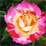# Moran'I Expected value

3299
0
06-28-2012 05:10 AMNew Contributor II
Hi evereboy !

I have a mathemathical question.

The tool "Spatial Autocorrelation" use the value of -1/(n-1) with n = number of indexed relation. This formula is also found in wikipedia.

I have not found it in others sources. The formula proposed by Moran is slightly different -(nm-n-m)/(nm(nm-1)) with n an m index of entity

In the article from Anselin, he says that E(Ii) = Wij/(n-1)

The simple rule of addition of expected value say that, as I = SUM(Ii),
E(I) = -(Sum(Wij))/(n-1)

what do you think ? can you show how we derived -1/(n-1)
or is there a old mistake somewhere ?

Thanks !
Tags (1)
0 Replies